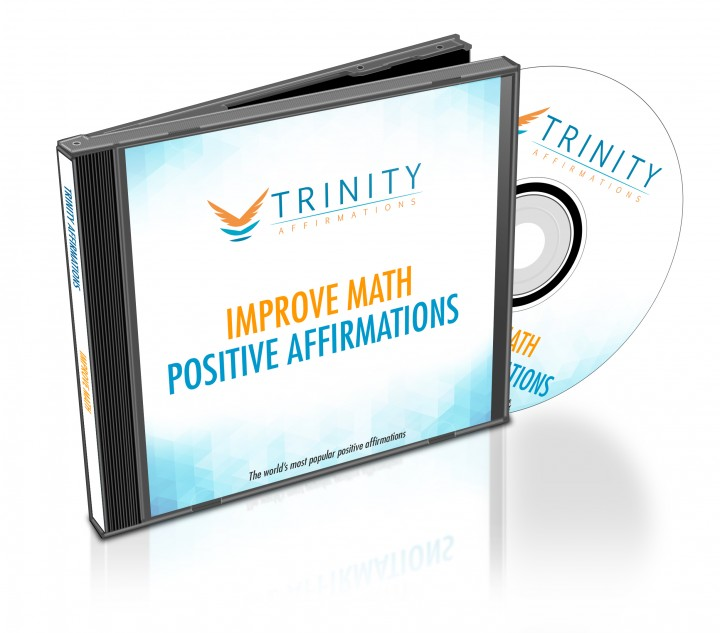# Improve Math Affirmations

Acquire the mindset of logical thinking people and become the kind of person who handles numbers easily using this transformational affirmation album.• Have you always been puzzled with maths formulae you studied?
• Do you find it difficult to calculate percentages?
• Has it always been easier to find something more interesting to do then really try hard to solve a math problem?
• Do you want help in learning to think like the math whiz you always wanted to be?

It is scientifically proven that there are several intelligences and that each of us has at least one. Unfortunately, the math intelligence was the first obvious and emerged as the most important. That is why our schools put the accent on learning it and consider only good mathematicians good students.

However, it doesn’t mean that if you are not good at maths you are less worthy. And it definitely doesn’t mean that you cannot be good at maths even if you are not born number smart. You can train yourself to calculate figures or solve math problems easily and quickly and this album can help you with that!

It was created for people like you who need help in focusing on the basic skills of a good mathematician. The first thing you have to change is your negative attitude towards the maths and this album will help you exactly with that. These powerful positive statements will show you that if you didn’t or don’t have a good grade in maths at school, it doesn’t mean you cannot be good at it. Quite the contrary!

# What to Expect

This album will:

• Help you easily remember all maths formulae. When you remember all the basic principles it will be easy for you to apply them to everyday situations. You will easily recognize the essential patterns you need and use them even for everyday functions, such as keeping your checkbook balanced or determining your gas mileage.
• Improve your study habits. These statements are particularly designed to develop areas of your brain that are responsible for mastering maths and they will help you improve your capability to learn, process information, stay focused and be motivated to study maths with dedication. They will drive you to achieve the most you possibly can.
• Re-program your mindset to acquire the ways of thinking that logical thinkers have. You will unlock your inner genius and your mind will function faster and more analytically. You will process maths problems effortlessly and solve everyday math problems quickly.

## Present Tense

• I am mathematically inclined
• I am great at math
• I love math
• I am a logical thinker
• I calculate figures easily
• I look for math problems to solve
• I process math problems effortlessly
• I am a mathematician
• I solve mathematical equations easily
• I solve everyday math problems quickly

## Future Tense

• I will be adept at math
• I am learning how to solve math problems
• I am developing a love for math
• I am getting faster at solving equations
• I am learning to solve math problems in my head
• I will look for math problems to solve
• I will be a mathematician
• My math skills are improving
• I will calculate answers flawlessly
• I will think logically

## Natural Tense

• Math comes easy to me
• My checkbook is always balanced
• Answering math problems feels natural to me
• Mathematics is part of who I am
• Solving equations comes naturally to me
• My mind works easily on math problems
• Everyday math problems are easy to solve
• Math is a part of my every day life
• Math is enjoyable
• Solving problems is fun for me